# 23

## Summary

### Factorization

The number 23 is a prime number.

### Properties and families

Property or family Parameter values First few numbers Proof of satisfaction/membership/containment
prime number ninth prime number 2, 3, 5, 7, 11, 13, 17, 19, 23, 29, 31, 37, 41, 43, 47, 53, 59, 61, 67, 71, 73, 79, 83, 89, 97, [SHOW MORE]View list on OEIS A natural numberis prime if and only ifis not divisible by any prime less than or equal to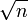. In this case, since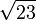is between 4 and 5, verifying primality requires verifying that 23 is not divisible by any prime up to 4, i.e., it is not divisible by 2 or 3.
safe prime (primesuch that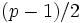is prime) fourth safe prime 5, 7, 11, 23, 47, 59, 83, 107, 167, [SHOW MORE]View list on OEIS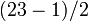equals 11, which is prime
Sophie Germain prime (primesuch that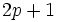is prime) fifth Sophie Germain prime 2, 3, 5, 11, 23, 29, 41, 53, 83, 89, [SHOW MORE]View list on OEIS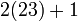equals 47, which is prime
factorial prime (prime that is of the form factorial) fifth factorial prime 2, 3, 5, 7, 23, 719, 5039, [SHOW MORE] View list on OEIS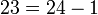and 24 equals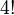## Polynomials

### Prime-generating polynomials

Below are some polynomials that give prime numbers for small input values, which give the value 23 for suitable input choice.

Polynomial Degree Some values for which it generates primes Input valueat which it generates 232 all numbers 1-10, because 11 is one of the lucky numbers of Euler. 42 all numbers 1-16, because 17 is one of the lucky numbers of Euler. 3

### Irreducible polynomials by Cohn's irreducibility criterion

By Cohn's irreducibility criterion, we know that if we write 23 in any base greater than or equal to 2, the corresponding polynomial is irreducible. We list here the irreducible polynomials of this type that are of degree at least two:

Base23 in baseCorresponding irreducible polynomial
2 101113 2124 113